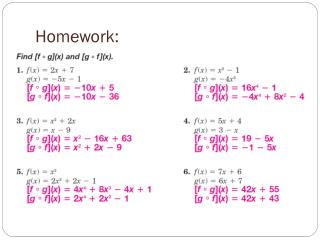# Homework: - PowerPoint PPT PresentationDownload PresentationHomework:

Homework:Download Presentation## Homework:

- - - - - - - - - - - - - - - - - - - - - - - - - - - E N D - - - - - - - - - - - - - - - - - - - - - - - - - - -
##### Presentation Transcript

1. Homework:

2. Warm-up: • Review 9.c adding and subtracting rational expressions • GET OUT YOUR 9.c NOTES!!!

3. Same denominator?

4. Example 1:

5. Different denominators? You must build a LCD!!! • Factor each denominator completely. • Multiply each fraction, top and bottom, by any factors from the other fraction that it is missing. • Write as one fraction BEFORE you do any multiplying out. • Multiply out numerator and collect like terms. • Factor numerator and reduce if possible.

6. Example 2:

7. Example 3:

8. Example 4:

9. You do some!

11. 8.d Find and Graph the Inverse of a Function or Relation [8.8] After this lesson you will be able to… • Find and graph the inverse of a function. • Determine whether 2 functions are inverses of each other.

12. What do you think of when you hear “inverse”?

13. Inverse Functions • An inverse function will take ananswer from the original function and “undo” it to give you back the x-value that produced the answer. • If original function is called ________ then the inverse is called _________.

14. Example 1: • Let’s pick an x-value and get an answer so we can test the inverse! • Put the answer into the inverse. • You should get the original x-value back!

15. Let’s test another!

16. Building your own inverse! • Replace f(x) with y (it is just easier to look at that way). • Switch the x and y in the equation. • Resolve the equation for y. • The result is the inverse. • Now check by using an x-value in the original!

17. Example 3: • Build the inverse. • Choose an x-value to use in the original, plug answer into inverse. Did it work? • Graph the original and it’s inverse on the same graph.

18. Example 4: • Build the inverse. • Choose an x-value to use in the original, plug answer into inverse. Did it work? • Graph the original and it’s inverse on the same graph.

19. WOW! • Inverse functions _______________________ over ____________________!!!

20. Homework: • HW ST 8.d